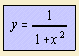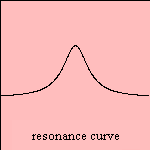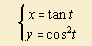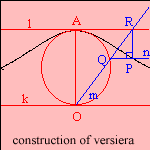# resonance curve

## cubic

last updated: 2004-04-11A well-known parameter equation for this curve is the following:Resonance occurs, when an external oscillation is exerted on a system, with a frequency in the neighborhood of a certain resonance frequency. An example is direct monochromatic light falling onto an atom. The intensity of the radiation, emitted by the atom has the form of a resonance curve, as function of the difference in frequency 1) (between external and resonance frequency).

One specimen of this class has been given special attentionsince the 17th century. We're talking about the versiera, which is constructed as follows.
Given a circle and two parallel lines k and l. Line k is a tangent to this circle in point O, l in point A.
Construct a line bundle m through O. This line m cuts the circle in Q, line l in R. Make now a line n parallel to l and m through Q. Then the point P of the curve is found by letting down a line down on n from point R.
In other words: P has the x-coordinate of point R and the y-coordinate of point Q.
It may be clear that not all resonance curves obey this construction rule.

The curve is:

The curve has been studied by Fermat (1666) and Grandi (1703).The Italian female mathematician Maria Gaetana Agnesi (1718-1799) wrote about the curve in her book 'Instituzioni analitiche (ad uso della gioventu italiana)' (1748).
She used the Latin word 'versoria' for the curve, meaning 'a rope that turns a sail'. This 'versoria' became the the Italian word 'la versiera', which means 'free to move'. But the translator of the book, the Englishman John Colson, translated the word to 'l'aversiera', the Italian word for 'witch'. So nowadays the curve is known as versiera or witch of Agnesi.

Other names referring to Agnesi are: Agnesi's cubic and (in French) agnésienne.

The curve is also a probability density function with the name of the Cauchy distribution.

The integral of the function is the arctangent.

notes

1) Given is a linear system, i.e. the system is being described by a linear differential equation. Further, the external force fluctuates in the form of a sine. Remember that the intensity of the light is proportional to the square amplitude. Then the given formula follows directly.
Wichmann 1967 p.109

../literature.html